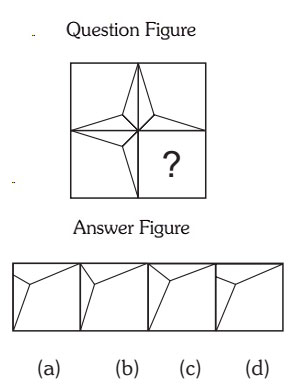#### Completion Of Incomplete Pattern

Direction: In each of the following questions, find out which of the answer figures (a), (b), (c) and (d) completes the figure?

1. Select the correct figure from the given Answer Figure that would complete the Question figure.1. Option (a) in Answer Figure.
2. Option (b) in Answer Figure.
3. Option (c) in Answer Figure.
4. Option (d) in Answer Figure.
##### Correct Option: C

Figure (c) will complete the figure if you check carefully.